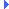FD.io VPP  v19.08-24-ge6a5712 Vector Packet ProcessingInclude dependency graph for load_balance.h:This graph shows which files directly or indirectly include this file:

Go to the source code of this file.

## Data Structures

The load-balance object represents an ECMP choice. More...

One path from an [EU]CMP set that the client wants to add to a load-balance object. More...

The FIB DPO provieds;. More...

## Macros

#define LB_NUM_INLINE_BUCKETS   4
The number of buckets that a load-balance object can have and still fit in one cache-line. More...

#define LB_HAS_INLINE_BUCKETS(_lb)   ((_lb)->lb_n_buckets <= LB_NUM_INLINE_BUCKETS)

## Typedefs

The load-balance object represents an ECMP choice. More...

One path from an [EU]CMP set that the client wants to add to a load-balance object. More...

Flags controlling load-balance creation and modification. More...

The FIB DPO provieds;. More...

## Enumerations

Flags controlling load-balance creation and modification. More...

## Functions

index_t load_balance_create (u32 num_buckets, dpo_proto_t lb_proto, flow_hash_config_t fhc)

void load_balance_set_bucket (index_t lbi, u32 bucket, const dpo_id_t *next)

void load_balance_set_urpf (index_t lbi, index_t urpf)

void load_balance_set_fib_entry_flags (index_t lbi, fib_entry_flag_t flags)

const dpo_id_tload_balance_get_bucket (index_t lbi, u32 bucket)

## Variables

The one instance of load-balance main. More...

The encapsulation breakages are for fast DP access. More...

## Macro Definition Documentation

 #define FOR_EACH_LOAD_BALANCE_ATTR ( _attr ) for (_attr = 0; _attr <= LOAD_BALANCE_ATTR_STICKY; _attr++)

Definition at line 92 of file load_balance.h.

 #define LB_HAS_INLINE_BUCKETS ( _lb ) ((_lb)->lb_n_buckets <= LB_NUM_INLINE_BUCKETS)

Definition at line 225 of file load_balance.h.

 #define LB_NUM_INLINE_BUCKETS   4

The number of buckets that a load-balance object can have and still fit in one cache-line.

Definition at line 56 of file load_balance.h.

Value:

Definition at line 87 of file load_balance.h.

## Typedef Documentation

Flags controlling load-balance creation and modification.

The load-balance object represents an ECMP choice.

The buckets of a load balance object point to the sub-graph after the choice is made. THe load-balance object is also object type returned from a FIB table lookup. As such it needs to represent the case where there is only one coice. It may seem like overkill to use a load-balance object in this case, but the reason is for performance. If the load-balance object were not the result of the FIB lookup, then some other object would be. The case where there was ECMP this other object would need a load-balance as a parent and hence just add an unnecessary indirection.

It is also the object in the DP that represents a via-fib-entry in a recursive route. Load-balance main

One path from an [EU]CMP set that the client wants to add to a load-balance object.

The FIB DPO provieds;.

• load-balancing over the next DPOs in the chain/graph
• per-route counters

## Enumeration Type Documentation

Flags controlling load-balance creation and modification.

Enumerator

Definition at line 82 of file load_balance.h.

Enumerator

Definition at line 95 of file load_balance.h.

Enumerator

Definition at line 183 of file load_balance.h.

## Function Documentation

 u8* format_load_balance ( u8 * s, va_list * args )

Definition at line 175 of file load_balance.c.Here is the call graph for this function:Here is the caller graph for this function:

 index_t load_balance_create ( u32 num_buckets, dpo_proto_t lb_proto, flow_hash_config_t fhc )

Definition at line 239 of file load_balance.c.Here is the call graph for this function:Here is the caller graph for this function:

inlinestatic

Definition at line 220 of file load_balance.h.Here is the caller graph for this function:

 const dpo_id_t* load_balance_get_bucket ( index_t lbi, u32 bucket )

Definition at line 339 of file load_balance.c.Here is the call graph for this function:Here is the caller graph for this function:

 static const dpo_id_t* load_balance_get_bucket_i ( const load_balance_t * lb, u32 bucket )
inlinestatic

Definition at line 229 of file load_balance.h.Here is the call graph for this function:Here is the caller graph for this function:

 flow_hash_config_t load_balance_get_default_flow_hash ( dpo_proto_t lb_proto )

Definition at line 193 of file load_balance.c.Here is the caller graph for this function:

Definition at line 68 of file load_balance.c.

 index_t load_balance_get_urpf ( index_t lbi )

Definition at line 329 of file load_balance.c.Here is the call graph for this function:

 int load_balance_is_drop ( const dpo_id_t * dpo )

Definition at line 272 of file load_balance.c.Here is the call graph for this function:Here is the caller graph for this function:

Definition at line 955 of file load_balance.c.Here is the call graph for this function:Here is the caller graph for this function:

Definition at line 602 of file load_balance.c.Here is the call graph for this function:Here is the caller graph for this function:

 u16 load_balance_n_buckets ( index_t lbi )

Definition at line 289 of file load_balance.c.Here is the call graph for this function:Here is the caller graph for this function:

 void load_balance_set_bucket ( index_t lbi, u32 bucket, const dpo_id_t * next )

Definition at line 256 of file load_balance.c.Here is the call graph for this function:Here is the caller graph for this function:

 void load_balance_set_fib_entry_flags ( index_t lbi, fib_entry_flag_t flags )

Definition at line 299 of file load_balance.c.Here is the call graph for this function:Here is the caller graph for this function:

 void load_balance_set_urpf ( index_t lbi, index_t urpf )

Definition at line 310 of file load_balance.c.Here is the call graph for this function:Here is the caller graph for this function:

 STATIC_ASSERT ( sizeof(load_balance_t)<= CLIB_CACHE_LINE_BYTES, "A load_balance object size exceeds one cachline" )

## Variable Documentation

The one instance of load-balance main.

Definition at line 56 of file load_balance.c.